# Early Hydration Kinetics of Cementitious Materials Containing Different Steel Slag Powder Contents

Early Hydration Kinetics of Cementitious Materials Containing Different Steel Slag Powder Contents

Xiaowei Cui Wen Ni* Chao Ren

Beijing Key Laboratory on Resource-oriented Treatment of Industrial Pollutants, University of Science and Technology Beijing, Beijing, 10083, China

Shaanxi Key Laboratory of Comprehensive Utilization of Tailings Resources, Shangluo University, Shangluo 726000, China

Corresponding Author Email:
niwen@ces.ustb.edu.cn
Page:
590-596
|
DOI:
https://doi.org/10.18280/ijht.340406
|
Accepted:
|
Published:
31 December 2016
| Citation

OPEN ACCESS

Abstract:

This study measures the hydration heat emission rate and quantity of cementitious materials containing 10%, 20%, 30% and 40% of steel slag (SS) powder at 298 K, calculates the kinetic parameters such as the reaction rate constant k, the degree of hydration α, and the order of reaction n according to the Krstulovic-Dabic hydration kinetics model, and discusses the influence of SS content over the hydration reaction and mechanism of cementitious materials. Results show that, with the increase in SS content, the hydration heat emission quantity decreases. The hydration process of cementitious materials containing different SS contents involves three stages, namely, nucleation and crystal growth (NG), interactions at phase boundaries (I), and diffusion (D). The Krstulovic-Dabic model can effectively simulate the hydration process of SS-based cementitious materials prepared with all-solid wastes. With the increase in the SS content, the hydration reaction induction period prolonged and the generation of hydration products is slowed down. At the early stage of hydration, NG is the controlling factor, which is gradually replaced by I as the hydration degree increases, and eventually by D when the reaction reaches the final stage.

Keywords:

Steel slag powder, Hydration kinetics, Hydration mechanism.

1. Introduction

Over the years, the rapid development of iron and steel metallurgy has aggravated the resource consumption, energy consumption, and waste discharge problems [1-3]. By conducting in-depth investigations in the field of industrial solid waste, researchers determined the pozzolanic activity of steel slag (SS), slag, tailings and other kinds of industrial solid waste, and used their findings to prepare building materials. Prepared with all-solid wastes, SS-based cementitous materials release considerable amount of heat during the hydration reaction. Researchers should explore the chemical reactions via by kinetics to reveal its microscopic mechanism dynamically. Merzouki  used the Knudsen model to analyze the influence of slag content, water-binder ratio and other factors on the hydration heat emission quantity of composite cementitious materials and determined that the model can accurately predict the hydration heat emission rate of composite cementitious materials with a low slag content. However, the model fails to explain the hydration reaction mechanism. Kolani  measured and analyzed the hydration heat emission quantity, the content of free and bound water, and the Ca(OH)2 content of composite cementitious materials, by using a multiphase hydration kinetics model. The results indicate that  slag replacement and temperature have a significant influence over the hydration degree of composite cementitious materials. Krstulovic  reported that the hydration reaction process of cement-based materials consists of three stages, namely nucleation and crystal growth (NG), interactions at phase boundaries (I) and diffusion (D). By using the Krstulovic-Dasic model, Yan Peiyu [7-9], Wang Qiang   et al. [10-14] discussed the hydration rate of cement-GGBSsteel slagprobed into the hydration kinetics of cement-based cementitious materials, and revealed that the reaction process simulated by the Krstulovic-Basic model  is consistent with the actual reaction process.

In this study cementitious materials were prepared with all-solid wastes such as SS, slag and desulfurized gypsum, which has not been reported. Then, the influence of SS content on the hydration heat emission rate and quantity and was analyzed the hydration kinetics and mechanism of cementitious materials were discussed.

2. The Hydration Kinetics Model of Cementitious Material

For ordinary chemical reactions, the rate constant $k(T)=A \cdot e^{-\frac{E_{2}}{R T}}$. Thus, the kinetic equation of a heterogeneous system at a defined temperature is expressed as :

$\frac{d_{\alpha}}{d_{t}}=k(T) \cdot f(\alpha)=A \cdot e^{-\frac{E_{a}}{R T}} \cdot f(\alpha)$(1)

where a is the degree of hydration reaction of the raw materials; t is the duration of the reaction; $f(\alpha)$ is the reaction mechanism function; T is the thermodynamic temperature; A is the pre-exponential factor; $T$ is the apparent activation energy; and R is the universal gas constant.

In the Krstulovic-Dabic model, the hydration reaction process of cement-based cementitious materials consists of three stages, namely, NG, I, and D. Similar to the hydration process of cement-based cementitious materials, the hydration process of cementitious materials prepared with all-solid wastes is also a heterogeneous reaction system involving the three stages of NG, I, and D. During the hydration reaction, the three stages occur simultaneously. Thus, every hydration reaction process has the following stages:

$\mathrm{NG} \quad \frac{d_{\alpha}}{d_{t}}=F_{1}(\alpha)=K_{1} n(1-\alpha)[-\ln (1-\alpha)]^{\frac{\mathrm{n}-1}{n}}$(2)

$\mathrm{I} \frac{d_{\alpha}}{d_{t}}=F_{2}(\alpha)=3 K_{2} R^{-1}(1-\alpha)^{2 / 3}$(3)

$\mathrm{D} \quad \frac{d_{\alpha}}{d_{t}}=F_{3}(\alpha)=3\left[K_{3} R^{-2}(1-\alpha)^{2 / 3}\right] /\left[2-2(1-\alpha)^{1 / 3}\right]$(4)

where $\alpha$ is the degree of hydration reaction of the raw material; t is the duration of the reaction; K1, K2 and K3 are the reaction rate constants; n is the order of reaction; and R is the universal gas constant.

Given that R is a constant,$K_{1}^{\prime}=K_{1}, K_{2}^{\prime}=K_{2} R^{-1},$ and $K_{3}^{\prime}=K_{3} R^{-2}$, are set can be used to derive the constant$K_{1}, K_{2}$ and $K_{3}$. Thus, as long as α is determined, the hydration reaction of cementitious materials can be simulated by the Krstulovic-Dabic model.

The hydration kinetics equation proposed by Knudson is introduced and expressed as :

$\frac{1}{Q(t)}=\frac{1}{Q_{\max }}+\frac{t_{50}}{Q_{\max }\left(t ~ t_{0}\right)}$(5)

to derive the following equations:

$\alpha=\frac{Q}{Q_{\max }}$(6)

$\frac{d_{a}}{d_{\mathrm{t}}}=\frac{1}{Q_{\mathrm{max}}} \cdot \frac{d Q}{d t}$(7)

where Q(t) is the hydration heat emission quantity at the time t of the reaction of the cementitious materials; Qmax  is the hydration heat emission quantity of the cementitious materials if the reaction duration is infinite; t50 is the time it takes for the hydration heat emission quantity to reach 50% of Qmax; and t0 is the end time of the hydration reaction induction period of the cementitious materials.

3. Experiment

Prepared with all-solid wastes, the cementitious materials are mixtures of three raw materials,namelySS, slag and desulfurized gypsum. The SS sampled from Wuhan Yuhua Steel Co., Ltd. in Hebei Province, China mainly contains C2S, C3S, C2F and RO (the solid solution of MgO, FeO, and MnO),  and a small amount of CaO. Figure 1 shows the XRD spectrum of SS. The slag is sampled from the blast furnace slag provided by Hebei Jintaicheng Building Materials Co., Ltd. The gypsum is selected from the desulfurized gypsum provided by Shijingshan Thermal Power Plant in Beijing. Table 1 lists the chemical composition of the main raw materials.

## 1.png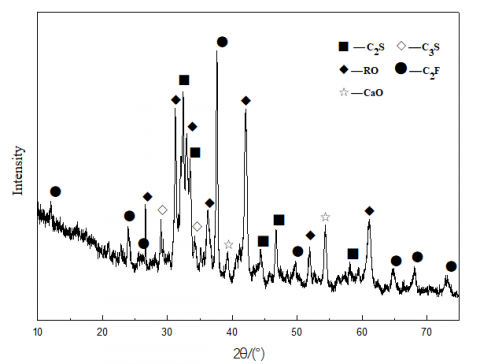Figure 1. XRD spectrum of SS

The SS, slag, and gypsum are ground until the specific surface area reaches 560, 580, and 640 m2/kg, respectively. The cementitious materials are prepared with different mix proportions according to the plan shown in Table 2.

Table 1. Chemical composition of the main raw materials (mass fraction, %)

 Material SiO2 Al2O3 Fe2O3 CaO MgO SO3 Na2O K2O TiO2 Steel slag 18.16 6.24 17.66 42.58 5.26 0.29 0.12 0.12 1.60 Blast furnace slag 26.15 13.49 2.96 41.41 10.09 0.83 0.27 0.35 1.67 Desulfurized gypsum 3.14 1.48 0.71 45.31 0.58 47.26 0.10 0.35 0.07

Table 2. Mix proportion of the cementitious materials (mass fraction, %)

 No. SS Slag Desulfurized gypsum a 10 78 12 b 20 68 12 c 30 58 12 d 40 48 12

The hydration heat emission rate and quantity of the cementitious materials are continuously measured for 200 h with Thermometric TAM Air eight-channel microcalorimeter. The water/binder ratio is 0.50 (mass ratio), and the test temperature is 25 °C.

4. Results Analysis

4.1 The pattern of hydration heat emission of the cementitious materials

Figures 2 and 3 show the hydration heat emission rate and quantity of the cementitious materials, respectively. The first exothermic peak of the cementitious materials usually appears 0.5 h after the materials have been mixed and stirred with water. During this moment, the cement is still being prepared. Thus, investigating the pattern of hydration heat emission of cementitious materials after the completion of pouring is more practical.

## 2.pngFigure 2. The hydration heat emission rate of the cementitious materials

## 3.png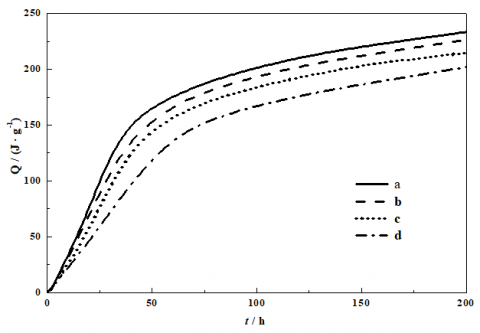Figure 3. The cumulative heat release of the cementitious materials

Figures 2 and 3 also show that the hydration reaction process of the cementitious materials prepared with all-solid wastes bears resemblances to that of cement. The process has five stages, i.e., preinduction, induction, acceleration, deceleration, and stabilization stages [15-16].  When the SS content is 10%, 20%, 30%, and 40%, the hydration reaction rate of the cementitious materials decreases with the increase in SS content. At the second exothermic peak, the maximum hydration heat emission rate is 10.91, 8.65, 6.92, and 3.80 J·g−1·h−1. At this moment, the hydration reaction has lasted for 19.7, 26.7, 35.5, and 40.5 h.. When the hydration reaction has lasted for 48 h, the hydration heat emission quantity of the cementitious materials in Groups a, b, c and d is 162.38, 149.71, 140.37, and 114.22 J·g−1, respectively.. Compared with Group a, the maximum hydration heat emission quantity of Groups b, c and d are 7.80%, 13.55%, and 29.66% lower.Figure 2, shows that the third exothermic peak appears at the SS content of 30% and becomes more prominent at the SS content of 40%. That is more AFt are converted to AFm in the hydration reaction as the C3A content in the cementitious materials prepared with all-solid wastes increases with the addition of SS .

The hydration induction stage of cementitious materials prepared with all-solid wastes requires nucleation control. Before the formation of crystal nucleus and the hydration products, Ca(OH)2 in the solution must reach a certain concentration . In the meantime, the Ca2+ concentration in the solution is affected by slag absorption. In the early stage of the reaction system, the pH and Ca2+ concentration of the reaction system increase rapidly with the increase in SS content, which is conducive to slag dissolution. However, if the SS content is excessively high, it is impossible for the small amount of slag to swiftly absorb Ca2+ in the solution. When the Ca2+ absorption rate of slag is slower than the Ca2+ dissolution rate of SS, the further hydration of the SS is suppressed, the hydration reaction rate of the cementitious materials is slowed down, the second exothermic peak is significantly delayed, and the maximum hydration heat emission rate and quantity are decreased.

4.2 Hydration kinetics analysis of the cementitious materials

The hydration heat data of the cemetitious materials of the experiment are substituted into Equation (5),  and the value of the maximum cumulative heat release Qmax of the hydration reaction of the cementitious materials is determined by linear fitting. Figure 4 shows the fitting of the maximum hydration heat emission quantity Qmax of the hydration reaction of the cementitious materials in Group a.

The degree of hydration α (t)is calculated by Equation (6). α (t) is substituted into Equation (2) to obtain ln [−ln(1 − α )] and ln(tt0). The kinetic parameters at the NG stage n and $K_{1}^{\prime}$ are obtained by linear fitting of ln [-ln(1 - α )] and ln(t - t0). Figure 5 shows the linear fitting of the kinetic parameters of the NG stage.

The kinetic parameters of stage I $K_{2}^{\prime}$and stage D $K_{3}^{\prime}$ are obtained in the similar manner and by the linear fitting with Equation (3) and Equation (4), respectively. On this basis, the reaction rates of NG, I, and D, i.e., F1(α), F2(α), and F3(α), respectively,can be acquired using Equations (2) to (4). In reference to the handling of Group A, Groups b, c and d are processed to obtain the corresponding kinetic parameters. Figure 6 shows the relationship between the degree of hydration α and the dα/dt, F1(α), and F2(α) of the hydration process of the cementitious materials in Groups a, b, c and d. Table 3 shows the kinetic parameters of the hydration process of the cementitious materials in each group.

As shown in Figure 6, F1(α), F2(α) and F3(α) exhibit good simulations of the hydration reaction process of the cementitious materials. Specifically, between 0.05 and $\alpha_{1}$, F1(α) is in good agreement with dα/dt; between $\alpha_{1}$ and $\alpha_{2}$, F2(α) is in good agreement with dα/dt; between $\alpha_{2}$ and 1, F3(α) is in good agreement with dα/dt. The hydration kinetics of all cementitious materials consists of the three stages of NG, I and D. Similar to that of cement, the hydration reaction of cementitious materials prepared with all-solid wastes is also a multiple, complex process controlled by a variety of factors. With $\alpha_{1}$ and $\alpha_{2}$ denoting for the transition points from NG to I and from I to D, respectively, the dominant factor and reaction mechanism are observed to change with the reaction time. With relatively few hydration products, NG is the dominant factor at the early stage of the reaction. The hydration products gradually increase and the ion migration becomes difficult with time. In this case, the dominant factor of the hydration reaction changes to I or D [7, 15] .

## 4.png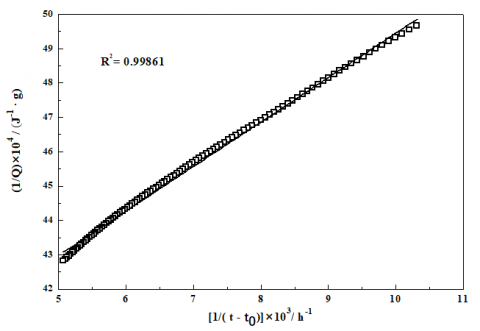Figure 4. Determination of the maximum quantity of heat emission Qmax by linear fitting

## 5.png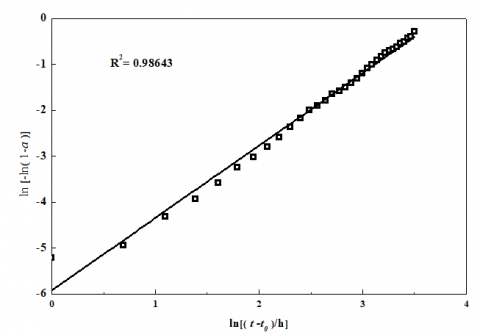Figure 5. Determination of the kinetic parameters of stage NG by linear regression

## 6a.png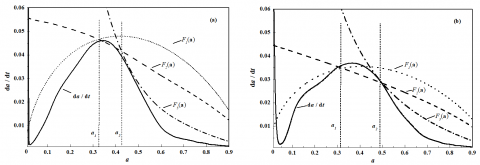## 6b.png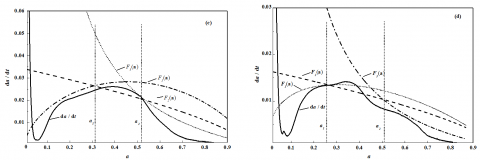Figure 6. Hydration rate curves of the cementitious materials

As shown in Table 3, the order of reaction n gradually decreases with the increase in SS content, an evidence for the influence of additional SS content on the formation of hydration products of the cementitious materials. With the increase in the SS content, $K_{1}^{\prime}$ and $K_{2}^{\prime}$ initially increase and then decrease, indicating that stage I of the hydration reaction of the cementitious materials is significantly affected by the proportion between SS and slag. With a low activity at the early stage, the dissolution of SS at a low content leads to the rapid increase in pH of the liquid phase, and the high concentration of Ca2+. In this case, the slag is rapidly activated, which accelerates the hydration reaction. When the SS content is relatively high, the proportion of slag in the system is reduced correspondingly. Given that the small amount of slag cannot rapidly absorb Ca2+ in the solution, the further hydration of the SS is suppressed, the hydration heat emission rate of the entire system is slowed down, and the reaction processeds to stage I at the low hydration level. This conclusion is also verified by the gradual decrease in $\alpha_{1}$. Moreover, $K_{3}^{\prime}$ increases with the SS content. The main reason for the increase is that the number of hydration reaction products is reduced at all stages of the reaction because of the increase in SS content, resulting in larger porosity in the system , lower resistance to ion diffusion, and delayed initiation of the diffusion mechanism. The silicate minerals in SS gradually hydrate and increase the Ca2+ concentration in the system with time.  In this case, the slag activity is significantly stimulated. The ensuing pozzolanic reaction absorbs Ca2+ to produce the C-S-H gel and AFt, which in turn stimulates SS dehydration. The synergistic effect of the two processes elevates the degree of hydration of the system, and the cementitious materials are no longer dominated by stage I when the degree of hydration is high, but by stage D. This finding is proven by the gradual increase in $\alpha_{2}$.

Table 3. The kinetic parameters of the hydration process of the cementitious materials

 No. n $K_{1}^{\prime}$ $K_{2}^{\prime}$ $K_{3}^{\prime}$ $\alpha_{1}$ $\alpha_{2}$ Process a 1.6760 0.0234 0.0167 0.0042 0.325 0.425 NG-I-D b 1.552 0.0430 0.0177 0.0044 0.313 0.491 NG-I-D c 1.438 0.0275 0.0134 0.0048 0.304 0.517 NG-I-D d 1.564 0.0179 0.0062 0.0054 0.254 0.519 NG-I-D

4.3 Micromorphology of the hydration reaction

Figure 7 shows the SEM images of neat paste specimens made from the cementitious materials in Groups a, b, c and d at the water/binder ratio of 0.20 after 1 day.

As shown in Figure 7, the hydration reaction has occurred in the cementitious materials of both groups after 1day. Numerous hydration products have been formed with C-S-H gel and the columnar AFt crystals intertwined. The hardened slurry network structure is substantially formed [19-21].

## 7a.png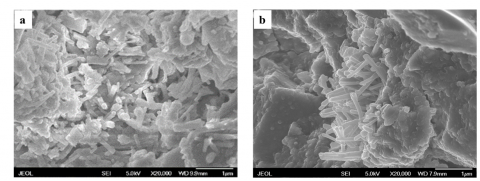## 7b.png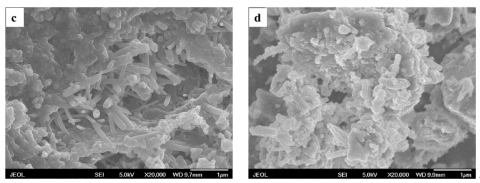Figure 7. SEM images of pastes curd for 3d

At the early stage of hydration reaction, the hydration of SS produces the C-S-H gel and Ca(OH)2 and rapidly increases the alkalinity of the system [22-23] . Being the other main raw material in the cementitious materials, slag mostly encompasses a large number of silicon tetrahedrons and aluminum oxide tetrahedrons, and possesses potential reactivity. In an alkaline environment, the weak Al–O bond of slag breaks first and produces H3AlO42- , which in turn reacts with Ca2+ in the alkaline solution to produce the C-S-H gel. The comparison of Figures 7(a), 7(b), 7(c), and 7(d) shows that with the increase in SS content, the amount of C-S-H gel and AFt produced by the cementitious materials containing after 1 day are significantly reduced. AFt in the hydration products of the cementitious materials in Group a surpasses its counterpart in Group d in terms of thickness, quantity and shape completeness . This finding also verifies the correctness of the hydration kinetics analysis.

5. Conclusions

(1) With the increase in SS content, the hydration heat emission rate of the cementitious materials is slowed down, the second exothermic peak is delayed, and the hydration heat emission quantity is significantly reduced. A significant third exothermic peak appears when the SS content exceeds 30%.

(2) The hydration reaction of the cementitious materials prepared with all-solid wastes can be classified into three stages, namely, NG, I, and D. The Krstulovic–Dabic model can ensure good simulations of the hydration reaction process of SS-based cementitious materials prepared with all-solid wastes.

(3) With the increase in the SS content, the hydration reaction induction time of the cementitious materials prepared with all-solid wastes is prolonged. At the early stage of hydration, NG is the controlling factor, which is gradually replaced by I as the hydration degree increases, and eventually by D when the reaction reaches the final stage.

Acknowledgment

Authors would like to acknowledge National High-tech R&D Program of China (863 Program) (No.2012AA062405) and the support from by Comprehensive Utilization of Tailing Resources Key Laboratory of Shaanxi Province (2014SKY-WK001, 2014SKY-WK017).

References

 Z. H. Zhang, J. L. LIAO, J. T. JU, “Treatment process and utilization technology of steel slag in China abroad,” Journal of Iron & Steel Research, vol. 25, no. 7, pp. 1-4, 2013.

 A. Mirandola, E. Lorenzini, “Energy, environment and climate: from the past to the future,” International Journal of Heat and Technology, vol. 34, no. 2, pp. 159-164, June, 2016. DOI: 10.18280/ijht.340201.

 I. Boulaoued, I. Amara, A. Mhimid, “Experimental determination of thermal conductivity and diffusivity of new building insulating materials,” International Journal of Heat and Technology, vol. 34, no. 2, June, 2016, pp. 325-331. DOI: 10.18280/ijht.340224.

 Merzouki T, Bouasker M, Khalifan, “ Contribution to the modeling of hydration and chemical shrinkage of slag-blended cement at early age,” Constr Buil Mater, vol. 44, pp. 368-380, 2013. DOI: 10.1016/j.conbuildmat.2013.02.022.

 Kolani B, Buffo -Lacarrère L, Sellier A, “Hydration of slag-blended cements,” Cem Concr Compos, vol. 34, no. 9, pp. 1009-1018, 2012. DOI: 10.1016/j.cemconcomp.2012.05.007.

 Krstulovic R, Dabic P, “A conceptual model of the cement hydration process,” Cem Concr Res, vol. 30, no. 5, pp. 693-698, 2000. DOI: 10.1016/S0008-8846(00)00231-3.

 P. Y. YAN, F. Zheng, “Kinetics model for the hydration mechanism of cementitious materials,” Journal of the Chinese Ceramic Society, vol. 34, no. 5, pp. 555-559, 2006. DOI: 10.14062/j.issn.0454-5648.2006.05.009.

 P.Y.Yan, Q. Wang, “Effect of high temperature curing on the early hydration characteristics of a complex binder containing steel slag,” Journal of Tsinghua University (Science and Technology), vol. 49, no. 6, pp. 790- 793, 2009. DOI: 10.16511/j.cnki.qhdxxb.2009.06.020.

 P. Y. Yan, Q. H. Zhang, “Compressive strength development of complex binder containing fly ash or quartz powder,” Journal of the Chinese Ceramic Society, vol. 35, no. 3, pp. 263-267, 2007. DOI: 10.14062/j.issn.0454-5648.2007.03.001.

 Q. Wang, P.Y. Yan, “Hydration properties of basic oxygen furnace steel slag,” Construction and Building Materials, vol. 24, no. 7, pp. 1134-1140, 2010. DIO: 10.1016/j.conbuildmat.2009.12.028.

 Q. Wang, P.Y. Yan, X. M. Kong, “Compressive strength development and microstructure of cement-asphalt mortar,” Journal of Wuhan University of Technology-Materials Science Edition, vol. 26, no. 5, pp. 998-1003, 2011. DIO: 10.1007/s11595-011-0351-9.

 Q. Wang; P. Y. Yan, J. W. Yang, “Comparison of hydration properties between cement-GGBS-fly ash blended binder and cement-GGBS-steel slag blended binder,” Journal of Wuhan University of Technology(Materials Science Edition), Vol.29 No.2, pp. 273-277, 2014. DOI: 10.1007/s11595-014-0907-6.

 Q. Wang, “Cementitious properties of steel slag and its role in the hydration and hardening process of complex binder,” Ph.D. dissertation, Dept. Tsinghua University, Beijing, China, 2010.

 Q. Wang, P. Y. Yan, S. Han, “The influence of steel slag on the hydration of cement during the hydration process of complex binder,” Science China Technological Sciences, vol. 54, no. 2, p. 388- 394, 2011. DOI: 10.1007/s11431-010-4204-0.

 F. H. Han, D. M. Wang, P. Y. Yan, “Hydration kinetics of composite binder containing different content of slag or fly ash,” Journal of the Chinese Ceramic Society, vol. 42, no. 5, pp. 613-620, 2014. DOI: 10.7521/j. issn. 0454 —5648. 2014. 05.10.

 Knudsen T, “On particle size distribution in cement hydration,” in Paris, Proceeding of 7th International Congress on the Chemistry of Cement, 1980, pp. 170-172.

 N. G. S, Justnes H., “Influence of dispersing agents on the rheology and early heat of hydration of blended cements with high loading of calcined marl,” Cement & Concrete Composites, no. 60, pp. 123- 134, 2015. DOI: 10.1016/j.cemconcomp.2015.04.007.

 TU K. J. X. Liu, K. Deng, “Study of the hydration behavior of steel slag and steel slag cement complex powders,” Journal of Beijing University of Chemical Technology: Natural Science, vol. 42. no. 1, pp. 62- 68, 2015.

 Y. T. Jia, “The hydration mechanism of the BFS and FA cement based materials,” Ph.D. dissertation, Dept. Southeast University, Jiangsu, China, 2005.

 Q. Wang, P. Y. Yan, “Early hydration characteristics and paste structure of complex binding material containing high-volume steel slag,” Journal of The Chinese Ceramic Society, vol. 36, no. 10, pp. 1406-1410, 2008. DOI: 10.14062/j.issn.0454-5648. 2008.10.027.

 X. Y. Huang, Z. Wang, Y. Liu, W. Hu, W. Ni, “On the use of blast furnace slag and steel slag in the preparation of green artificial reef concrete,” Constr Build Mater, no. 112, pp. 241-246, 2016. DOI: 10.1016/j.conbuildmat.2016.02.088.

 D.L.C. Angulski, R. D. Hooton, “Influence of curing temperature on the process of hydration of super sulfated cements at early age,” Cement Concrete Res. no. 77, pp. 69-75, 2015.

 A., Islam U. J. Alengaram, M. Z. Jumaat, II. Bashar, “The development of compressive strength of ground granulated blast furnace slag-palm oil fuel ash-fly ash based geopolymer mortar,” Mater Design, no. 56, pp. 833-841, 2014. DOI: 10.1016/j.matdes.2013.11.080.

 H. Wu, W. Ni, X.W. Cui, Wang S, “Preparation of concrete sleeper using hot steaming steel slag with low autogenous shrinkage,” Transactions of Materials and Heat Treatment, vol. 35, no. 4, pp. 7-12, 2014. DOI:  10.13289/j.issn.1009-6264.

 K. Wang, C. Qian, R. Wang, “The properties and mechanism of microbial mineralized steel slag bricks,” Constr Build Mater, no. 113, pp. 815-823, 2016. DOI: 10.1016/j.conbuildmat.2016.03.122.